The mechanical system shown consists of a cylinder and a block. The moment of inertia of the cylinder is J with respect to its center bearing, and both the displacement z and the angle of rotation theta are measured from their respective equilibrium positions. At its bearing (point O) the cylinder experiences a viscous moment Md and a restorning moment Mr (not shown in the figure), both in the opposite direction of the roational motion. The inputs of the system are the driving force and moment u1=F and u2=M. The required outputs are y1=displacement of the block, y2=angle of rotation of the rotor, y3=force in the spring, y4=viscous moment at the bearing, and y5=acceleration of the block. (a) Obtain the state space representation of the system. (b) For the given inputs: F=15S(t) N and M(t)=-50u(t) Nm with zero initial conditions, plot the outputs for 10 s. (c) For the given inputs shown in the figure with zero initial conditions, plot the outputs for 10s. (d) For the given inputs plot the outputs for 12 s: F(t)=10sin12t N and M(t)=-40cos8t Nm.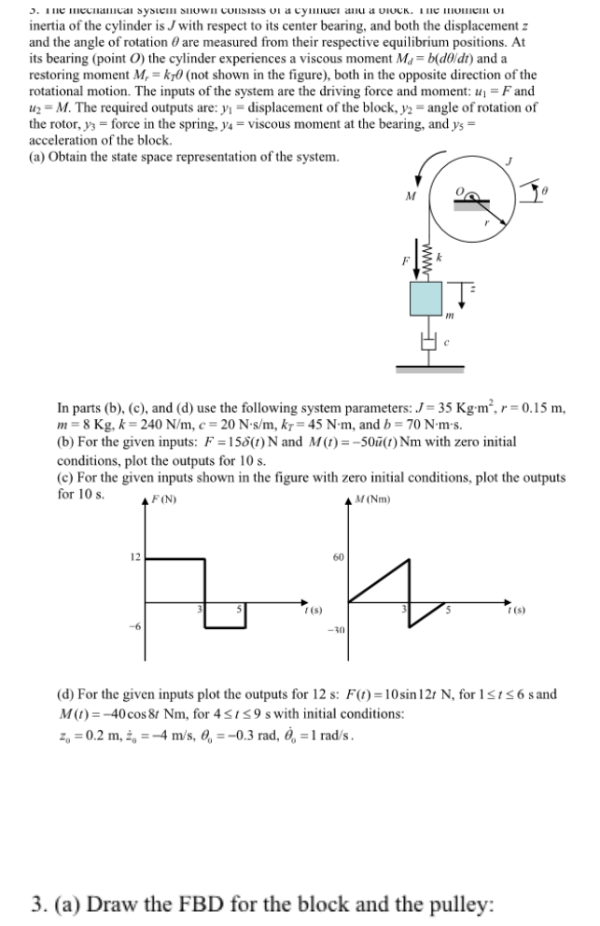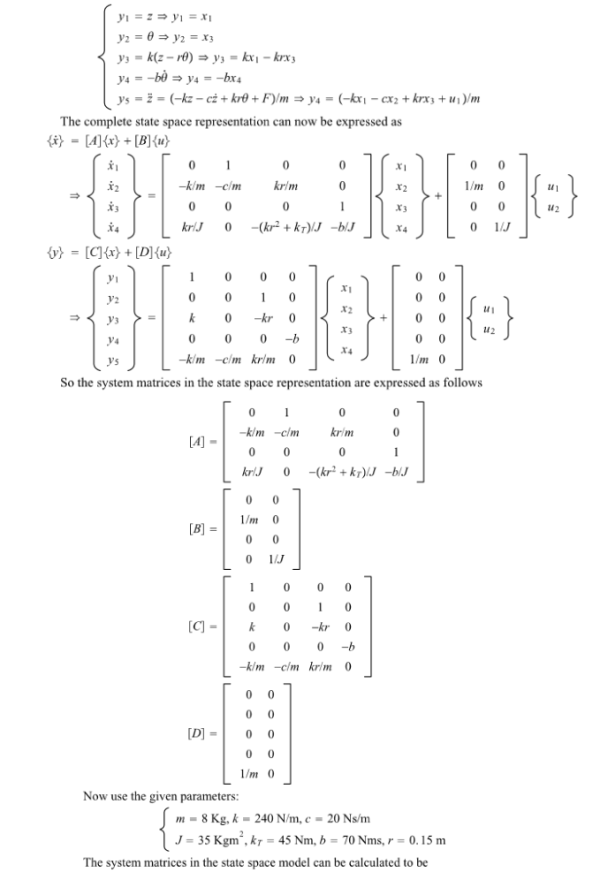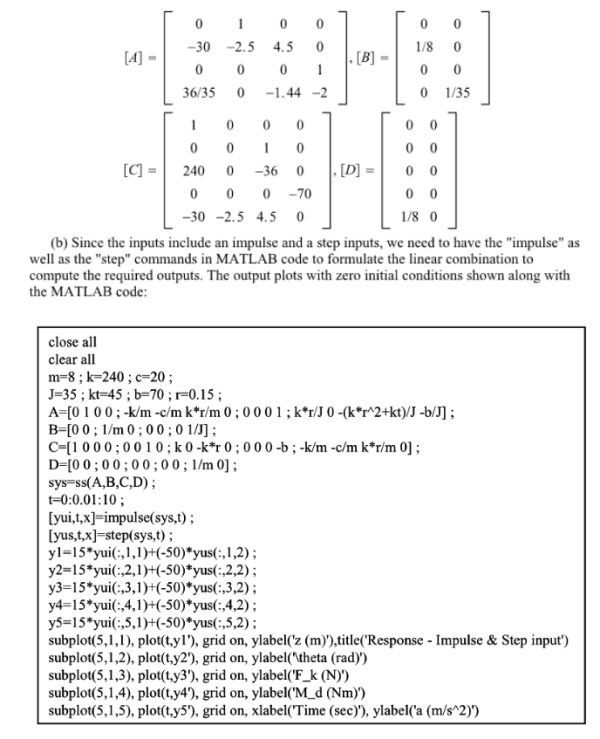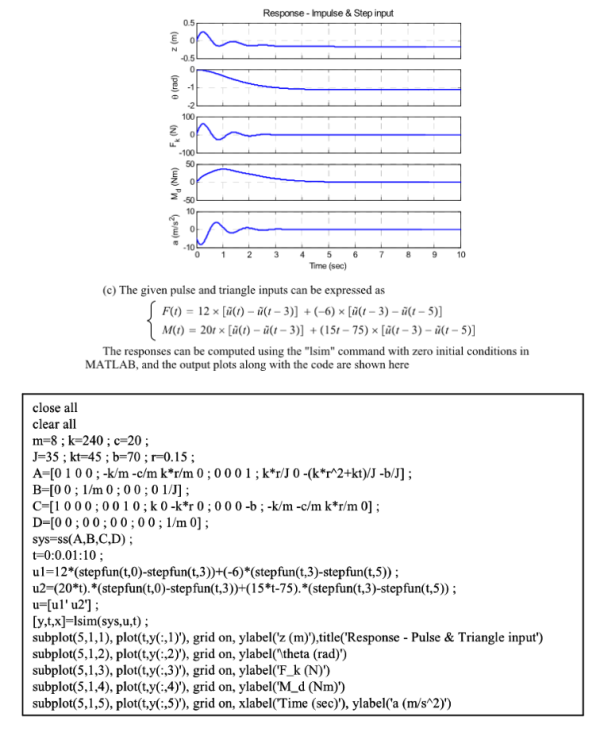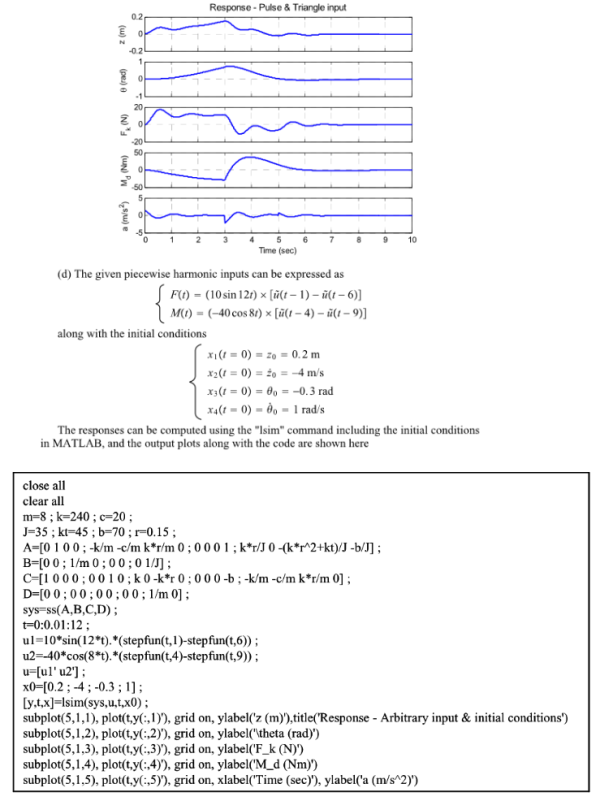The mechanical system shown consists of a cylinder and a block. The moment of inertia of the cylinder is J with respect to its center bearing, and both the displacement z and the angle of rotation theta are measured from their respective equilibrium positions. At its bearing (point O) the cylinder experiences a viscous moment Md and a restorning moment Mr (not shown in the figure), both in the opposite direction of the roational motion. The inputs of the system are the driving force and moment u1=F and u2=M. The required outputs are y1=displacement of the block, y2=angle of rotation of the rotor, y3=force in the spring, y4=viscous moment at the bearing, and y5=acceleration of the block. (a) Obtain the state space representation of the system. (b) For the given inputs: F=15S(t) N and M(t)=-50u(t) Nm with zero initial conditions, plot the outputs for 10 s. (c) For the given inputs shown in the figure with zero initial conditions, plot the outputs for 10s. (d) For the given inputs plot the outputs for 12 s: F(t)=10sin12t N and M(t)=-40cos8t Nm.

System Dynamics Page 2 dynamics dynamics dynamics dynamics dynamics dynamics dynamics System dynamics Page 3